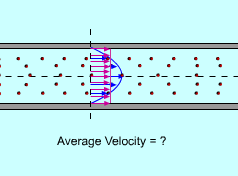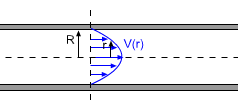Ch 9. Integrate Application Multimedia Engineering Math Area BetweenCurves Volume Work Average Value
 Chapter 1. Limits 2. Derivatives I 3. Derivatives II 4. Mean Value 5. Curve Sketching 6. Integrals 7. Inverse Functions 8. Integration Tech. 9. Integrate App. 10. Parametric Eqs. 11. Polar Coord. 12. Series Appendix Basic Math Units Search eBooks Dynamics Fluids Math Mechanics Statics Thermodynamics Author(s): Hengzhong Wen Chean Chin Ngo Meirong Huang Kurt Gramoll ©Kurt GramollMATHEMATICS - CASE STUDY IntroductionBlood flowing in a VesselVelocity of the Blood is a Function of r Amanda works at a biomedical engineering lab. For a particular project, a sensor needs to be installed in a blood vessel at a location where the blood velocity has the same magnitude as the average velocity of the whole vessel cross-sectional area. What is known: The vessel can be considered as a cylindrical tube with a radius of 0.08 mm and a length of 20 mm. The blood in the vessel is considered as a fully developed laminar flow with a velocity function as v(r) = [P / (4μl)] (R2 - r2) where P is the pressure difference between the ends of the vessel, P = 400 Pa, μ is the viscosity of the blood, μ = 10x10-3 kg/(m-s), R is the radius of the vessel, and l is the length of the vessel. Questions Where does the blood velocity have the same magnitude as the average velocity of the whole cross-sectional area of the vessel? What is the average velocity of the whole vessel cross-sectional area? Approach Use the Mean Value Theorem for Integrals.